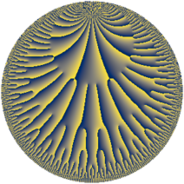# Properties

 Label 25.34.aLevel $25$ Weight $34$ Character orbit 25.a Rep. character $\chi_{25}(1,\cdot)$ Character field $\Q$ Dimension $51$ Newform subspaces $6$ Sturm bound $85$ Trace bound $2$

# Related objects

## Defining parameters

 Level: $$N$$ $$=$$ $$25 = 5^{2}$$ Weight: $$k$$ $$=$$ $$34$$ Character orbit: $$[\chi]$$ $$=$$ 25.a (trivial) Character field: $$\Q$$ Newform subspaces: $$6$$ Sturm bound: $$85$$ Trace bound: $$2$$ Distinguishing $$T_p$$: $$2$$

## Dimensions

The following table gives the dimensions of various subspaces of $$M_{34}(\Gamma_0(25))$$.

Total New Old
Modular forms 85 54 31
Cusp forms 79 51 28
Eisenstein series 6 3 3

The following table gives the dimensions of the cuspidal new subspaces with specified eigenvalues for the Atkin-Lehner operators and the Fricke involution.

$$5$$Dim.
$$+$$$$24$$
$$-$$$$27$$

## Trace form

 $$51q - 56142q^{2} - 49445066q^{3} + 216841931222q^{4} - 14954277834678q^{6} + 113491809006458q^{7} - 406680168722760q^{8} + 96256649142625233q^{9} + O(q^{10})$$ $$51q - 56142q^{2} - 49445066q^{3} + 216841931222q^{4} - 14954277834678q^{6} + 113491809006458q^{7} - 406680168722760q^{8} + 96256649142625233q^{9} - 172169568271932318q^{11} - 1266207059723367952q^{12} + 1987504964122341174q^{13} + 18899750821698508764q^{14} + 879700625083823019346q^{16} + 253705940786565344958q^{17} - 1410213206838192403286q^{18} - 390372905430751829530q^{19} + 11770445021243563369272q^{21} - 13464751189533983039144q^{22} - 88497491964705425934786q^{23} - 127789899298794594701490q^{24} - 26274024222069617946708q^{26} - 65931887505368933271260q^{27} + 1225073295064679301855776q^{28} - 155733873994737304036770q^{29} + 4296784774232547937486392q^{31} - 20297052434774866460462112q^{32} + 6082814658188234370787088q^{33} - 79537473971829713292461386q^{34} + 502709537677566846709202376q^{36} - 29118377652523422686673242q^{37} - 468462593114733778981689240q^{38} - 58530593290348812104560884q^{39} + 1459620753857820321591874572q^{41} + 1577002543368372696868932576q^{42} - 1475165303134337029316393106q^{43} + 640734787758797873868009954q^{44} + 2412815863785963896560623412q^{46} - 1526890651470833573802978942q^{47} - 26551235505425991057878326336q^{48} + 43359288688289268950333051207q^{49} + 100944740947462046763058226622q^{51} + 74546850515849387893402346728q^{52} - 129695812483567983847363790466q^{53} - 44463054142511860227782717730q^{54} + 615675163504177847241161852220q^{56} + 22175228408354623481404503880q^{57} - 19130093473398680760339154660q^{58} - 157660121658345455994894227940q^{59} - 297615246081660048246618174618q^{61} - 111432702032104669754030974464q^{62} + 1062788499606414102138922558314q^{63} + 1329815900975589561853349280322q^{64} - 994003352042502703327620303846q^{66} - 1198604889673461043715653991542q^{67} + 3452647663630989852843111975576q^{68} + 6836872807117437106547522290776q^{69} - 10859965557860618164915074089388q^{71} - 2299052831003508463274321421480q^{72} + 14440161824825659727868325976214q^{73} - 7860035618629488649317948460536q^{74} - 5047075990436304950388998006510q^{76} - 15193964629343892533632225024944q^{77} + 26884831908732328676034365694128q^{78} + 26578470462212288473571361327380q^{79} + 81155238975678532213362079652151q^{81} + 66580629236453077141160050419476q^{82} - 74547772948728256764442937694546q^{83} - 210001561981374752909421532111116q^{84} + 516980715961619981653263536096952q^{86} + 116159356744453612431399958360420q^{87} - 704961534025861282168642650964320q^{88} + 503770637817306119427284994876540q^{89} - 168466585609583480036671291733908q^{91} + 435439371265928097003268971463008q^{92} + 350609465666898243241143005104728q^{93} + 52656013686268209439219599588464q^{94} - 3047070860761489674711007915742658q^{96} - 1333574093387027687680300909316042q^{97} + 820588450412584719889391015754306q^{98} - 1886466912309808910998781706013644q^{99} + O(q^{100})$$

## Decomposition of $$S_{34}^{\mathrm{new}}(\Gamma_0(25))$$ into newform subspaces

Label Dim. $$A$$ Field CM Traces A-L signs $q$-expansion
$$a_2$$ $$a_3$$ $$a_5$$ $$a_7$$ 5
25.34.a.a $$2$$ $$172.457$$ $$\mathbb{Q}[x]/(x^{2} - \cdots)$$ None $$121680$$ $$-37919880$$ $$0$$ $$67\!\cdots\!00$$ $$+$$ $$q+(60840-\beta )q^{2}+(-18959940+312\beta )q^{3}+\cdots$$
25.34.a.b $$5$$ $$172.457$$ $$\mathbb{Q}[x]/(x^{5} - \cdots)$$ None $$-30472$$ $$14988714$$ $$0$$ $$65\!\cdots\!58$$ $$+$$ $$q+(-6094+\beta _{1})q^{2}+(2997861+296\beta _{1}+\cdots)q^{3}+\cdots$$
25.34.a.c $$6$$ $$172.457$$ $$\mathbb{Q}[x]/(x^{6} - \cdots)$$ None $$-147350$$ $$-26513900$$ $$0$$ $$-1\!\cdots\!00$$ $$+$$ $$q+(-24558-\beta _{1})q^{2}+(-4418958+\cdots)q^{3}+\cdots$$
25.34.a.d $$11$$ $$172.457$$ $$\mathbb{Q}[x]/(x^{11} - \cdots)$$ None $$-9393$$ $$-80330849$$ $$0$$ $$-2\!\cdots\!58$$ $$+$$ $$q+(-854+\beta _{1})q^{2}+(-7302793-124\beta _{1}+\cdots)q^{3}+\cdots$$
25.34.a.e $$11$$ $$172.457$$ $$\mathbb{Q}[x]/(x^{11} - \cdots)$$ None $$9393$$ $$80330849$$ $$0$$ $$21\!\cdots\!58$$ $$-$$ $$q+(854-\beta _{1})q^{2}+(7302793+124\beta _{1}+\cdots)q^{3}+\cdots$$
25.34.a.f $$16$$ $$172.457$$ $$\mathbb{Q}[x]/(x^{16} - \cdots)$$ None $$0$$ $$0$$ $$0$$ $$0$$ $$-$$ $$q+\beta _{1}q^{2}+(52\beta _{1}+\beta _{9})q^{3}+(4553207930+\cdots)q^{4}+\cdots$$

## Decomposition of $$S_{34}^{\mathrm{old}}(\Gamma_0(25))$$ into lower level spaces

$$S_{34}^{\mathrm{old}}(\Gamma_0(25)) \cong$$ $$S_{34}^{\mathrm{new}}(\Gamma_0(1))$$$$^{\oplus 3}$$$$\oplus$$$$S_{34}^{\mathrm{new}}(\Gamma_0(5))$$$$^{\oplus 2}$$